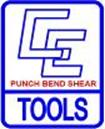Change MM to Inch  divide by 25.4   Inch to MM X 25.4   CM to Inch divide by 2.54 Inch to CM X 2.54
 Calculating Weights:  Round Steel Bars  Dia. X 2 * 2.67 = weight of 1’ Sheet/Rectangle Steel  T X L X W X Weight Density.  Weight Density:  Tool Steels, CRS, 41## =.283 Stainless= .___    Aluminum =CE TOOLING Formula’s-Conversions  of Fractions, Size & WeightChange Lbs.=Pounds to Kg.=Kilograms   Inch  X .45359237 = Kg. Kg.  Divided by .45359237  Inch to CM Multiply X 2.54Mark Up Price by specific percent.   Example: Mark up a \$200 item by 15%  \$200 / .85 = \$235.294   Determine list price if given Net price and told your getting 15% discount.  \$235.294 X .85
 CONVERSIONS
 MATERIAL GAGE EQUIVALENT CHART ANSI Gage 35 30 25 22 20 18 16 14 12 11 10 Decimal Equivalent .0075 .012 .02 .03 .035 .048 .06 .075 .105 .119 .135 ANSI Gage 9 8 7 6 5 4 3 Decimal Equivalent .15 .165 .18 .195 .209 .225 .24
 Coil Length in feet  =  Coil Weight                 (thickness)(width)(.2900)(12) MS=.29   SST=___       Alum=___